# Introduction to the frequency response of linear filters

by Craig Stuart Sapp
18 March 2003

This introduction to frequency response should help you to understand the relationship between spectrum and transfer functions as well as ways of calculating the behavior of arbitrary linear filters.  The transfer function of a filter is calculated by taking the z-transform of the difference equation for the filter and solving for Y/X as you will see demonstrated below. You will also get an overview of the methods used to evaluate the behaviour of a linear filter by examining the transfer function in various ways.  A complete plot of the transfer function input and output would be a four-dimensinoal plot which cannot be understood visually by humans.  Therefore, many plotting methods in 2, 2.5, and 3 dimensions for the transfer function are necessary to visualize the complete behavior of a linear filter.

### Frequency response of the averaging filter.

An averaging filter can be described with the following difference equation:
y[n] = (x[n] + x[n-1])/2
It would be interesting to know how this filter affects input signal frequencies.  This Mathematica notebook gives an overview of how to determine the effects of any linear filter; in other words, how to look at the spectrum changing properties of the filter.
Y/X = (1+1/z)/2

To determine how the averaging filter affects frequencies, you must first convert the difference equation into the following equation by taking the z-transform of the difference equation:
Y = (X + Xz^-1)/2
There is a lot of mathematics underlying the z-transform process, but you only need to know a few rules to take the z-transform of a difference equation:
(1) convert small y (output signal) and small x (input signal) to Y and X respectively
(2) convert [n] to z^0, [n-1] to z^-1, [n-2], z^-2, etc.
(3) all other parts of the difference equation will remain the same (such as the divide by 2 factor).

Now that you have the z-transformed difference equation, you need to solve the equation in terms of Y/X:
Y/X = (1+z^-1)/2
This form of the z-transformed difference equation is called the transfer function.  Everything you need to know about the frequency altering effects of the filter are contained in this equation.  Usually you will have to use a computer from this point on to calculate the effects of the filter on a signal unless working with a very simple filter such as the averaging filter.

In the following Mathematica function definition, the transfer function is duplicated.  The name of the Mathematica function is AverageTF which stands for the averaging filter's transfer function:

``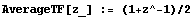``

The variable z in the transfer function is a two-dimensional number.  If you are familiar with cartesian plots with an x-axis and a y-axis, you can understand an analogy between these types of plots and the transfer function (as well as z).  In a cartesian plot, the x-axis is the dependent variable usually shown on the horizontal axis as given in the example cartesian plot below.  The function displayed on the vertical y-axis is the value x^2.  The x-axis is called the domain of the plot, and the y-axis is called the range of the plot.

``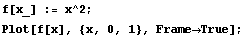``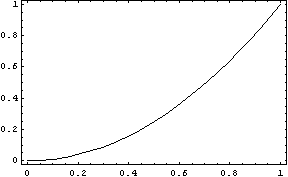So, think of the x-axis in the plot above like the input to a filter.  The y-axis would then be the output of the filter.  This is, by analyogy, the meaning of the transfer function.  The z value is functioning exactly like x in the plot above.  The z value is the domain of our transfer function plot.  Note that the z value is two dimensional (since I told you), therefore instead of calling it the z-axis, the domain of the transfer function is called the z-plane.  This is the origin of the z-plane term used in filter theory: it is the two-dimensional domain of a four-dimensinoal plot.

The range of the transfer function plot describes what happens to a z value when it goes through the filter.  This output range is typically
called the w-plane.  Like the z values, w values are two dimensional.

Of course, there is a bit of a problem in drawing a four-dimensional plot.  Therefore to visualize the transfer function, it has to be displayed usually as a spectrum (2 dimensional) or a pole-zero diagram (2.5 dimensions).  In a computer-based program like Mathematica, it is also easy
to generate a 3-D picture of the transfer function (projected into 2 dimensions, of course), but it would still be difficult to draw a four-dimensional plot (projected into 2 dimensions).

Pole-zero diagrams solve the visualization problem by displaying only the z-plane.  Then there are two special values of the w-plane which are superimposed onto the z-plane (where they are generated on the z-plane).  If the output of a filter goes to zero, then a circle is drawn over the value in the z-plane which caused that zero.  If the output of the fitler explodes to infinity, then an x is used to mark the location where this happened on the z-plane (x = pole).

Look again at the transfer function for the averaging filter:
w = (1 + 1/z)/2
For what values of z will the output number w become zero?  There is exactly one number: when z is equal to -1:
w[-1] = (1 + 1/(-1))/2
w[-1] = (1-1)/2
w[-1] = 0/2
w[-1] = 0
For what values of z will the output number of w become infinity?  There is exactly one number: when z is equal to 0:
w = (1 + 1/0)/2
1/0 is infinity, in case you do not know.  Adding anything to infinity is still infinity, and dividing by any number (except infinity of course) is still infinity.

We now know where the transfer function goes to zero (z=-1) and where it goes to infinity (z=0).  Now we have enough information to
draw the pole-zero diagram for the transfer function of the averaging filter.

``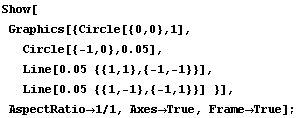``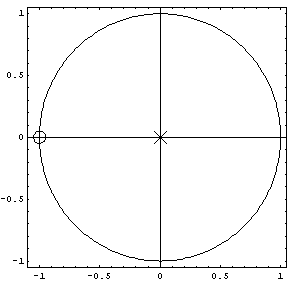The x-axis of the z-plane is called the real-axis of the z-plane because the real numbers are represented by it.  The y-axis of the z-plane is the imaginary axis of the z-plane where imaginary numbers are represented (imaginary numbers are scaled values of the square root of minus one and not literally imaginary!).  Now keep in mind that the pole-zero diagram is 2.5 dimensions.  You clearly see the two dimensions of the z-plane which is the domain of the transfer function.  You do not see any part of the w-plane which is the domain of the plot (like the y-axis in a two-dimensional plot).  Instead, you see marks on the z-plane where the w-plane values are either zero or inifinity.  Think about this a while so that you can understand the meaning of the following plots.

With Mathematica or another similar computer program it is easy to plot 3 dimensional plots.  This means that we can see a little more of the transfer function plot in a single diagram.  The z-plane and w-plane both represent complex numbers.  Complex numbers can be described in two general ways, either in cartesian coordiates  (as in the pole-zero plot above) or in polar coordinates (which describes numbers in terms of angle and magnitude).  When you are viewing a spectrum of a soundfile in two dimensions, you are viewing one dimension each of the z and w axes.  The x-axis of a standard spectrum plot is the angle of the complex number in the z-plane plot.  The y-axis of a standard spectrum plot is the magnitude of the numbers in the w-plane.

The following plot expands the dimensions of the pole-zero diagram.  The z-axis is the same as the pole-zero diagram in the plot above.  Now, instead of marking the locations of zeros and infinities where they occur, the third dimension of the plot will display the magnitude of the w-plane value even if it is not zero or inifinity.  Examine the following plot and try to see the correspondence with the pole-zero diagram above.  The z-axis is the "floor" of the 3D plot, and the height above the floor is the magnitude of the w-plane value.  You are looking at the plot from the left side of the plot-zero diagram with the zero right in the center of the front edge of the following plot.  The peak in the middle of the plot
corresponds to the infinity at z=0.  The pole is truncated, but in actuality the mountain in the middle of the plot has an infinte height at z=0.

``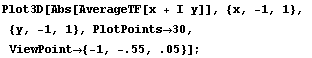``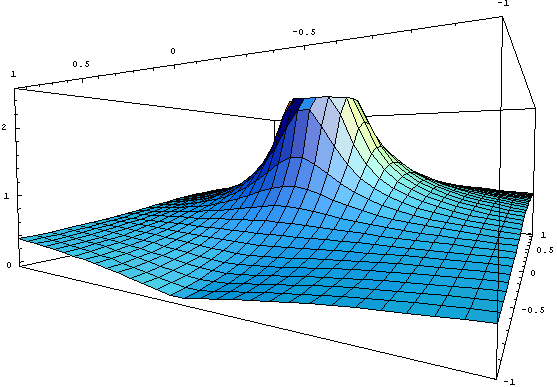It is not common to see this type of plot for the transfer function for a few reasons. (1) this plot is very difficult to draw by hand, and (2) the locations of the poles and zeros in the w-plane are the only important pieces of information necessary to describe the filter.

Look at the other side of the z-plane magnitude plot.  Notice that there is no dip down to zero on the other side of the mountain.  In fact, at z=1, the magnitude of w is exactly 1 (right in the middle of the front of the picture).

``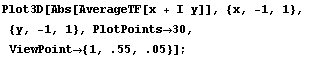``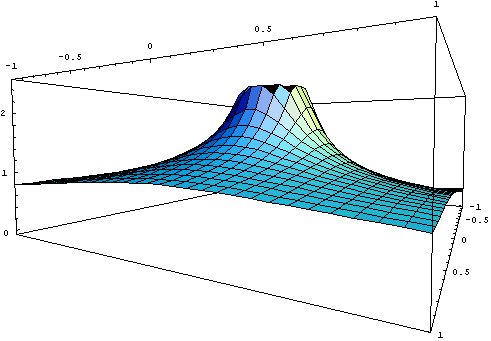Now, let's examine the relationship between the above z-plane magnitude plots and the more traditional spectrum plots of sound.

The vertical axis of the 3D plots above are exactly the same as the y-axis in traditional spectrum plots.  The x-axis of spectrum plots is a semi-circular path on the z-axis.  The radius of the circle for the path is one.  The angle starts at 0 which is where z=1 and then rotates counter-clockwise to 180 degrees where z = -1.  Notice that the circle which the tradional spectrum traverses is shown in the pole-zero diagram above.
This circle is called the unit circle because it has a radius of one (i.e., one unit).   Go back and look at the pole-zero diagram to see this circle.  The traditional spectrum starts at the right-most point on the circle in the pole-zero diagram and then rotates counter-clockwise to the left-most point on the circle.

The process of generating the spectrum can be thought of as taking a cookie-cutter in the shape of a circle.  Imagine cutting the magnitude z-plane plot above with a circular cookie cutter.  Now examine the surface of the cookie you have just cut.  It will look like this:

``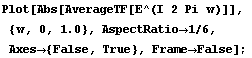``The point where the edge of the top of the cookie goes to zero is the point on the z-plane where the w value is zero.  This occurs at z=1 which is at the front of the first magnitude z-plane plot.  The back of the plot where z=-1 is the highest part of the edge of the cookie where the w value is 1.

Due to mathematics, real signals -- such as audio-- only need to be plotted half-way around the cookie.  One reason is that the second half of the circumference will always be a mirror image of the first part.  The other reason is that the frequencies of the second half will be aliased onto the first half.  Now we are ready to create the averaging filter's spectrum:

``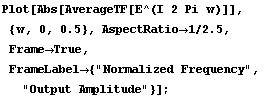``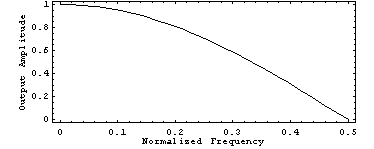This spectrum plot shows how the amplitude of each input sinewave will be affected by the averaging filter, given that the input amplitude of each sinewave was 1.0.  Notice that a 0 Hz signal does not lose any amplitude going through the filter.  A sample 0 Hz signal is the sequence:
1, 1, 1, 1, 1, 1, 1, 1, 1, 1, 1, 1, 1...
The output from the averaging filter is:
..., 1, 1, 1, 1, 1, 1, 1, 1, 1, 1, 1, 1, ...
Notice that the output signal amplitude is identical to the input signals amplitude: both are 1.

Now notice that the spectrum of the averaging filter goes to zero at 0.5 normalized frequency.  This is equivalent to 1/2 of the sampling rate, which is also called the Nyquist frequency.  A sample signal at this frequency is:
1, -1, 1, -1, 1, -1, 1, -1, 1, -1, 1, -1, 1, -1...
The output from the averaging filter for this frequency is:
..., 0, 0, 0, 0, 0, 0, 0, 0, 0, 0, 0, 0, 0, 0.
Notice that the amplitude of the input signal at the Nyquist frequency was 1, but the output amplitude for the signal was 0.

The x-axis of the spectrum above was from 0.0 to 0.5.  This is a measurement of distance around the unit circle.  To convert the spectrum x-axis plot to frequency, the x-axis just needs to be scaled by the sampling rate frequency so that the far right of the spectrum goes up to 1/2 of the sampling rate:

``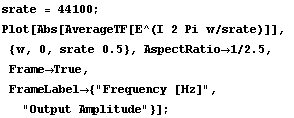``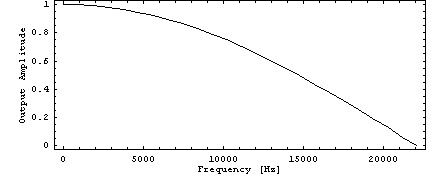It is customary to display the magnitude of a spectrum in decibels instead of units of amplitude, so the final spectrum plot for the averaging filter will look like this:

``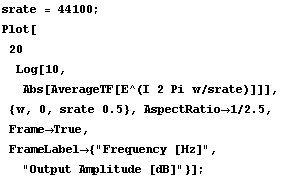``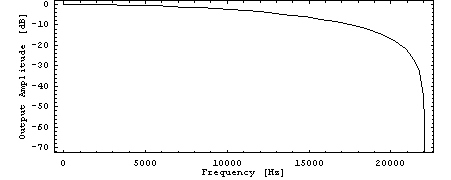Now remember that the 3D plot of the magnitude of the output of the filter was plotted over the z-plane.  There is one more dimension which is usually not paid much attention to in music since it is not as audible as magnitude changes in the filter: phase.  When processing audio on the computer, phase is usually just as important as magnitude, however.  Here is a plot of the phase of the filter in 3D.  The z-plane is the floor of the plot, and the height above the floor represents the phase of the filter.  In this picture you are looking from behind the pole, and the zero is in the center of the far edge of the plot

``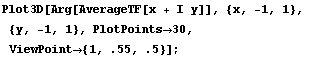``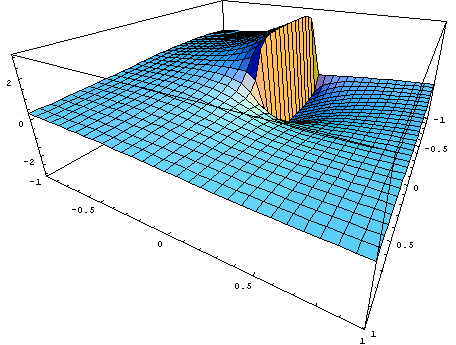The phase plot which is similar to the spectrum will look like this:

``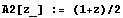``
``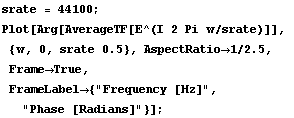``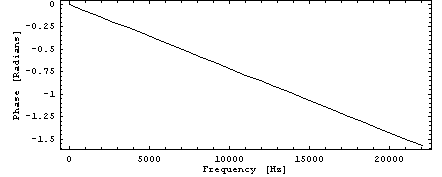This phase plot gives useful information about the filter.  In the case of the averaging filter, notice that the phase plot is a straight line.  This is true of all feedforward linear filters.  The slope of the line indicates the amount of delay a frequency took in going through the filter.  In this case the delay was a constant value for all frequencies.  Each frequency took 1/2 of a sample to pass through the filter.  Take for example the Nyquist frequency, the phase in this case is:

``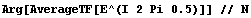``
``

This is equivalent to -Pi/2:

``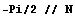``
`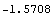`

Since the Nyquist frequency takes 2 samples to cycle, the delay of Pi/2 as it goes through the filter is equal to 1/2 of a sample delay.

You have now seen all of the important visualizations of the filter response for the averaging filter.  The domain of the response is the two-dimensional z-plane, and the range of the response is also two-dimensional.  Displaying four-dimensional plots are not possible, so 2, 2.5, and 3 dimensional plots are the only method of visualizing the transfer function which describes how the filter affects input signals.

### Frequency response of a one-pole feedback filter.

The averaging filter is a special case of the one-zero linear filter.  Another filter which would be interesting to examine in terms of transfer functions is are the feedback filters where the output of the filter is recycled in the filter.  Here is the difference equation of a one-pole feedback filter:
y[n] = a x[n] + b y[n-1]
The z-transform of this difference equation is:
Y = a X - b Y/z
The transfer function is then:
Y/X = a/(1-b/z)
or    Y/X = az / (z - b)

````
``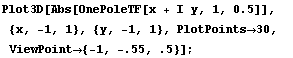``Notice that at z=0 there is a zero in the filter response rather than a pole as in the averaging filters magnitude plot.  The feedback gain was set to 0.5, which indicates the position of the pole on the real axis.  Here is a 3D plot which makes this easier to see:

``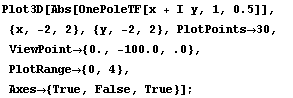``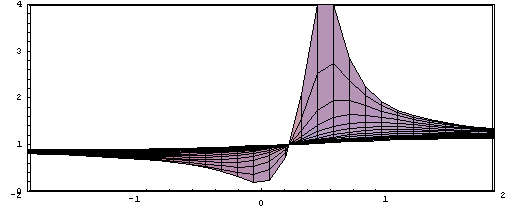If the feedback gain is set to -1, then notice how the pole moves to -1 on the real axis:

``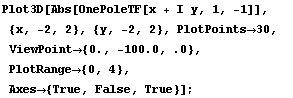``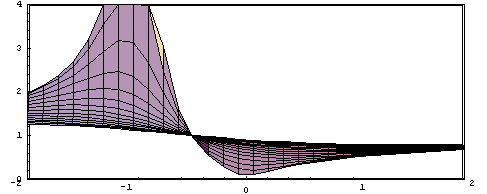Here is a plot of the magnitude spectrum which shows the elevation of the 3D magnitude plot when the feedback gain is 0.5:

````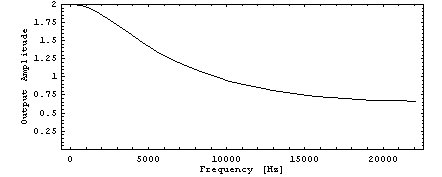Now look at the one-pole frequency response when the feedback gain is 1:

````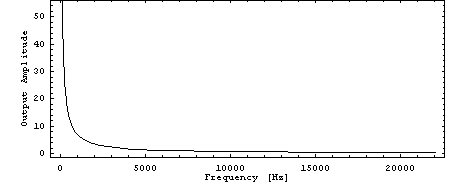As the frequency respose approaches zero Hz, the output gain of the filter approaches zero.  This is due to the pole seen in the magnitude response plots.  The pole is sitting right on the unit circle at z=1 in this case.  Therefore, any frequency energy entering the filter at this frequency will be trapped in the filter and eventually cause the filter to become unstable.  Here is what is happening in the filter when the feedback gain is 1.  Consider the 0 Hz signal:
1, 1, 1, 1, 1, 1, 1, 1, 1, 1, 1...
Put this signal through the filter which has the difference equation: y[n] = x[n] + y[n-1].  The feedback gain in this case is 1.0.  Here is what the output from the filter will be:
..., 1, 2, 3, 4, 5, 6, 7, 8, 9, 10, 11, 12, ...
Notice that every number going through the filter is summed with all of the previous inputs to the filter.  So you could think of this filter as the summation filter.  As long as you give the filter a balance of positive and negative numbers, it will not "blow up".  But if you continually give it only positive or only negative numbers, the output signal magnitude will become quite large.

Feedback filter phase responses are always very interesting, unlike feedforward filter phase responses which are always straight lines.  Here is the phase response of the one-pole filter when the feedback gain is set to 0.5:

``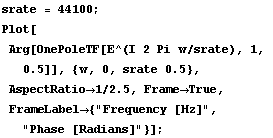``There are two general pieces of information you can extract from this plot. (1) the plot is not a straight line; therefore, the filter must contain a feedback term.  If the plot were straight, then you know that there are no feedback elements in the filter.  (2) you can tell that the frequencies between 5,000 Hz and 10,000 Hz are delayed longer in the filter than either frequencies close to 0 Hz or close to the Nyquist frequency.  At 0 Hz and the Nyquist frequency, there is no delay at all.

How much are the frequencies around 7,500 Hz delayed in the filter?  If you examine the slope of the phase response, you can tell:

``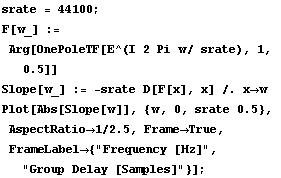``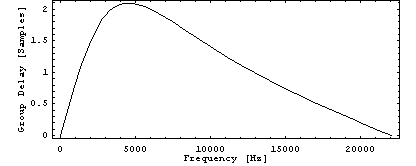The above plot shows what is called the group delay of the filter.  This plot for a feedback of 0.5 will have frequencies around 5000 Hz delayed by 2 samples as they travel through the filter.  For feedforward filters, the group delay is always zero, since the phase plots are always straight lines.

### Frequency response of a one-pole/one-zero  filter.

Just for fun, lets now look at a filter which has one feedback and one feedforward term:
y[n] = x[n] - x[n-1] + g * y[n-1]
The z-transform of this equation is:
Y = X - X/z + g Y/z
Solving for Y/X which is the transfer function:
Y/X = (1-1/z)/(1-g/z)
Simplifying:
Y/X = (z-1)/(z-g)
This filter is used to remove 0 Hz from audio, so it is often called the DC blocking filter.  And g is usually set to be about 0.99.  Let us
now look at how the filter effects the input signal by plotting various versions of the transfer function.

````

First, look at the 3D magnitude plot:

``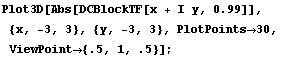``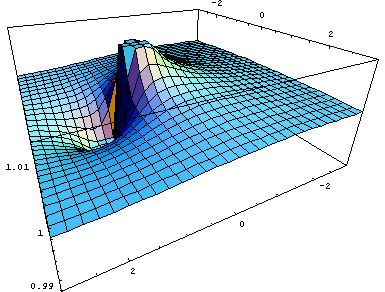It may be hard to see, but notice at z=0, there is neither a pole nor a zero.  The zero from the feedback part of the filter at z=0 is canceled exactly by the pole from the feedforward part of the filter at z=0.  Only the pole from the feedback part and the zero from the feedforward part are present in the transfer function plot.

Examining the magnitude spectrum plot makes it understandable as to why the filter is a DC blocking filter:

``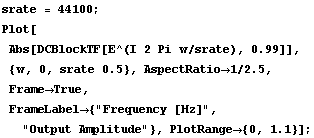``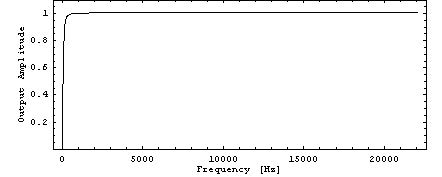Notice that for most of the frequency range from <1,000 to 22,000 the magnitude response is exactly (or nearly) 1.  At 0 Hertz, the output of the filter is exactly zero, and the is a sharp transition up to 1. Let's also view the filter amplitudes in decibel form:

``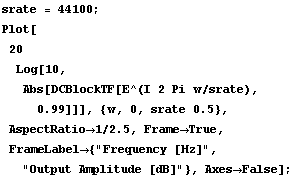``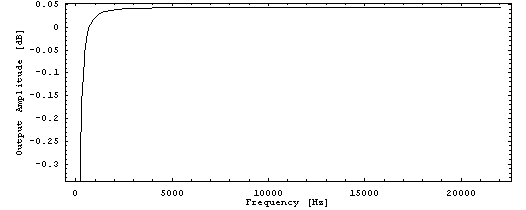Look at the lowest part of the graph at the transition region:

``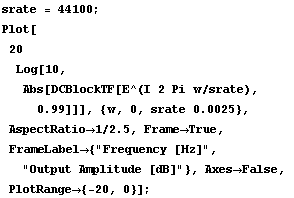``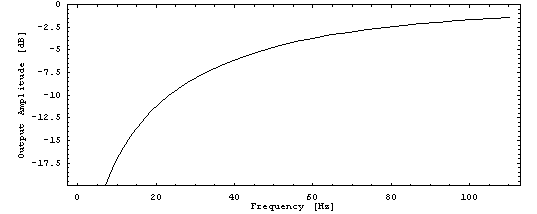This plot shows that with a feedback gain of 0.99, the DC blocking filter will significantly remove all frequencies below 10 Hz, but will also slightly affect the amplitude of the frequencies between 40 and 80 Hz.  Above 80 Hz, there will be very little audible difference in the output from the filter.  Between 40 and 80 Hz, there will perhaps be a slightly audible difference.

Here is the phase response of the DC Blocking filter:

``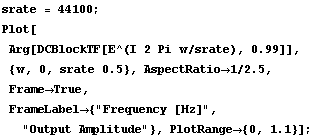``The group delay is particularly interesting to examine for filters.  Here is the group delay plot for this filter:

``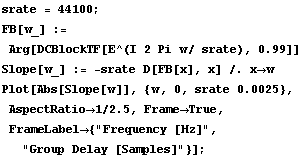``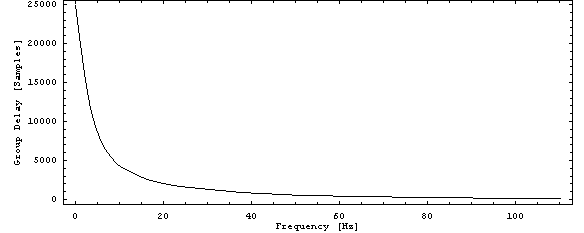In this case the delay of frequencies close to 0 Hz are 25, 000 samples, or almost 1/2 of a second! Of course, the lower the frequency the more it is suppressed from the output; therefore, this delay is not very audible, especially below 20 Hz where humans cannot hear.